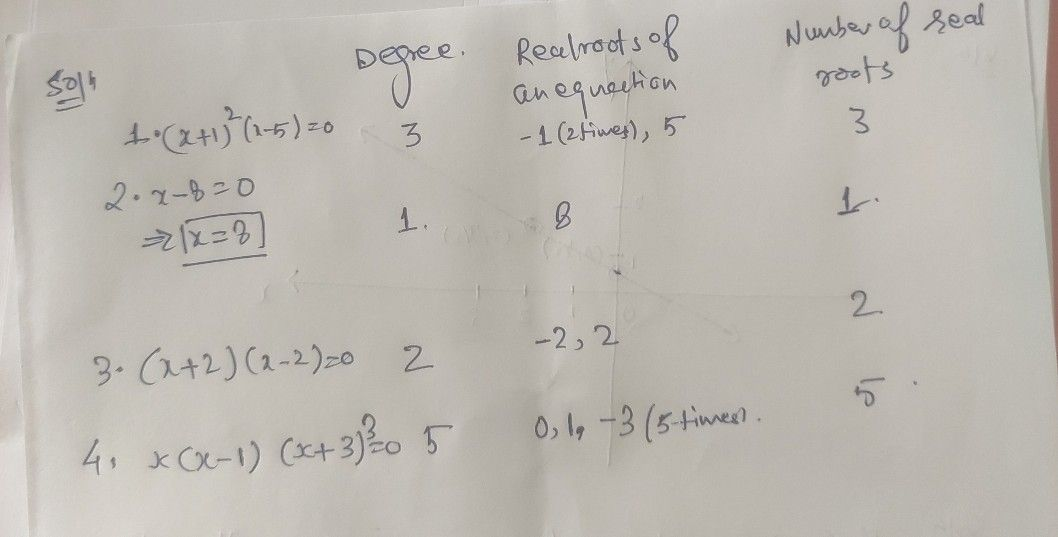Symbol
ProblemActivity 5 Some polynomials equations are given below.Complete the table and answer the questions that follow. $\left(1$ a root occurs twice, count it two times: if thrice.count it three times, and $50$ on). The first one is done for you. Polynomial Equation Degree Real Roots of an Number of Real Equation Roots $1.\left(x+1\right)^{2}$ $\left(x-5\right)$ $3$ $-1\left(2$ times); 5 $3$ $2.$ $x-8=0$ $3.$ $\left(x+2\right)\left(x-2\right)=0$ $4$ $0^{x\left(x-1\right)\left(x+3\right)^{3}}$